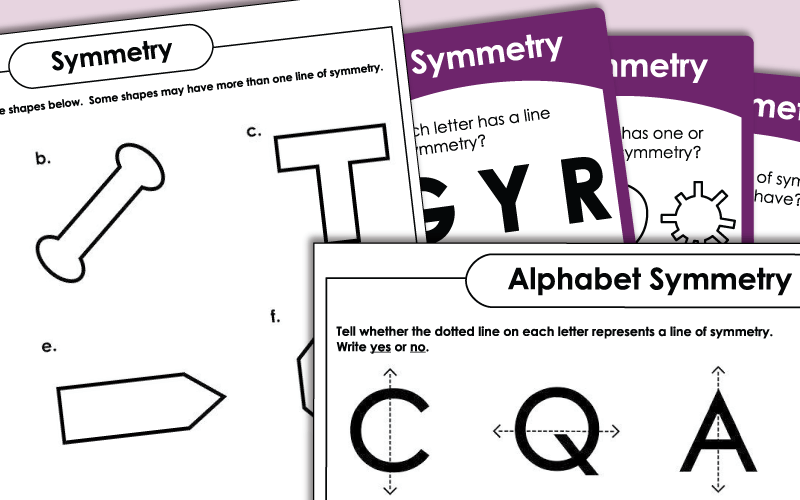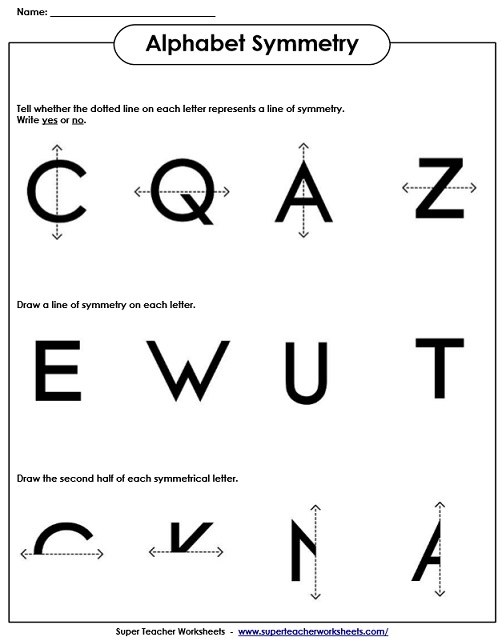# Symmetry Worksheets

Printable practice worksheets to help you teach and review symmetry.  Includes worksheets the require students to draw lines of symmetry, determine which pictures are symmetrical, and design symmetrical illustrations.Part 1: Tell whether the dotted lines on the shapes are lines of symmetry. Part 2: draw lines of symmetry on the shapes.Part 3: draw the second half of each symmetrical figure.
Draw the missing half of each symmetrical shape on the dotted graph paper.
Cut out these 12 large shapes and fold them on their lines of symmetry.
Determine whether the dotted line on each of the shapes is a line of symmetry.
Tell which of these houses are symmetrical and which ones aren't.
This file contains 20 symmetry task cards. Use them for small group instruction, learning centers, exit tickets, or classroom games.
Try to sketch four different symmetrical objects - a snowman, an alien, a sailboat, and a friend.  Use a red pen to draw the line of symmetry on each of your pictures.
Draw lines of symmetry of the capital letters.  Draw the second half of the symmetrical letters.
Color the pattern so that it makes a symmetrical design.
Examine the folded pictures and tell which shape you'll see when the paper is unfolded.
Add the lengths of the sides to find the perimeter of these symmetrical polygons. Requires advanced multiplication.
Also on Super Teacher Worksheets...
Geometry Worksheets

Learn about lines, angles, polygons, solid shapes, circles, and more!

Time Worksheets

Practice reading clocks and telling time.

Rounding Worksheets

Round numbers to the nearest ten, hundred, and thousand.

Pictures of Our Worksheets